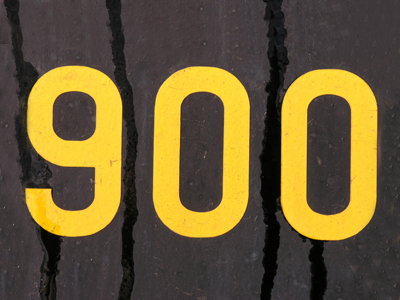The nearest 100 to 942 is 900.

# Rounding Numbers (Year 5/6)

In KS2 Maths children will look at rounding numbers up or down. In Years Five and Six children should be capable of rounding whole numbers to the nearest ten, hundred or thousand. In addition they will now look at the rounding of decimal numbers and fractions.

Knowing whether to round a number up or down is vital in the world of maths! It very much depends on whether the number is above or below half way. The same rules apply to rounding decimal numbers and whole ones. Just as the whole number 32 is rounded down to 30, the decimal number 3.2 is rounded down to 3. Fractions can also be rounded up or down. For example, 25 would be rounded down and 35 would be rounded up.

Test your knowledge of rounding numbers by playing this quiz.

1.
Which number is between 7,000 and 8,000?
650
6,500
7,500
8,500
7,500 is exactly halfway between 7,000 and 8,000 but would be rounded up rather than down
2.
Which is the nearest whole number to 1.36?
1
2
3
4
Decimals less than .5 are rounded down
3.
Which is the nearest whole number to 0.5?
0
1
2
3
When rounding to the nearest whole number, numbers ending in .5 are round up
4.
Which is the nearest 1,000 to 15,762?
10,000
15,000
16,000
17,000
15,762 is only 238 less than 16,000
5.
In which of these questions would you need to round the answer down?
A box holds 12 eggs. How many boxes will I need to hold 80 eggs?
Stickers are £3.75 each. I have £12. How many stickers can I buy?
There are 345 children and teachers going on a school trip. A bus holds 52. How many buses will be needed?
Hot dogs are £2.60. I have £10.40. How many hot dogs can I buy?
12 ÷ 3.75 = 3.2 so we have to round 3.2 down to 3. In the other questions we either round the answer up or leave it as it is
6.
Which is the nearest million to 3,798,631?
2,000,000
3,000,000
4,000,000
5,000,000
3,798,631 is 201,369 less than 4,000,000
7.
Which is the nearest 100 to 942?
800
900
1,000
1,100
942 is closer to 900 than to 1,000
8.
Which of these numbers would be rounded up to the nearest 10?
175
263
994
812
When rounding numbers to the nearest 10, numbers ending in 5 are rounded up
9.
Which is the nearest 1,000 to 2,845?
1,000
2,000
3,000
4,000
2,845 is much closer to 3,000 than it is to 2,000
10.
Which of these numbers would be rounded up to the nearest 1,000?
7,469
2,431
3,050
6,500
When rounding to the nearest 1,000, numbers ending in 500 are rounded up
You can find more about this topic by visiting BBC Bitesize - How to round decimal numbers

Author:  Amanda Swift